# Circle: Diameter, Radius, and Chord

A circle is formed by points that are same distance from a fixed point called the centre of the circle.

The centre of a circle is a point: A circle is named after its centre. If centre of a circle is point O, the circle would be named circle O or the circle with centre O.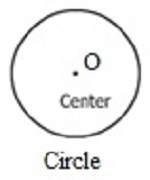The radius of a circle is any line segment connecting the centre of the circle to any point on the circle.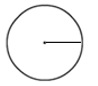The chord of a circle is a line segment joining any two points on the circle.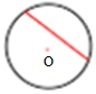The chord of a circle which passes through the centre of the circle is called the diameter of the circle. The diameter of a circle is the distance across a circle. The diameter is also the longest chord of a circle.Formula for the diameter of Circle

Diameter of a circle d = 2r, where r is the radius of the circle.

Find the radius and diameter of the following circle.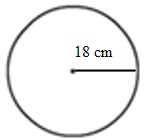### Solution

Step 1:

Given Radius r = 18 cm

Step 2:

So Diameter = 2r = 2(18) = 36 cm

Step 3:

So, Radius = 18 cm, Diameter = 36 cm

Identify the radius, diameter, and chord from given circle.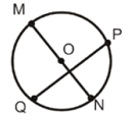### Solution

Step 1:

The chord is Chord QP

Step 2:

The diameter is Diameter MN